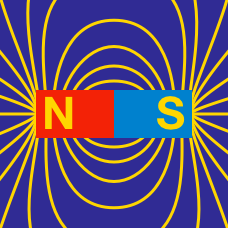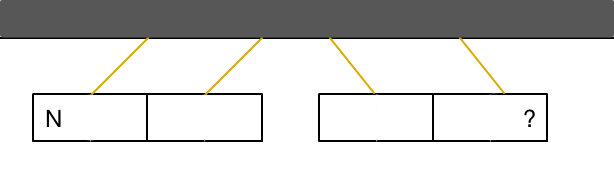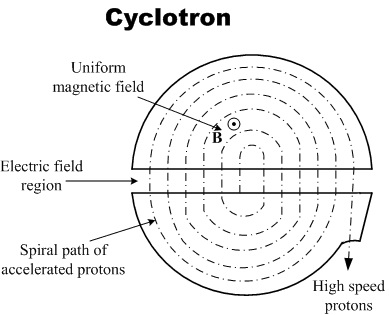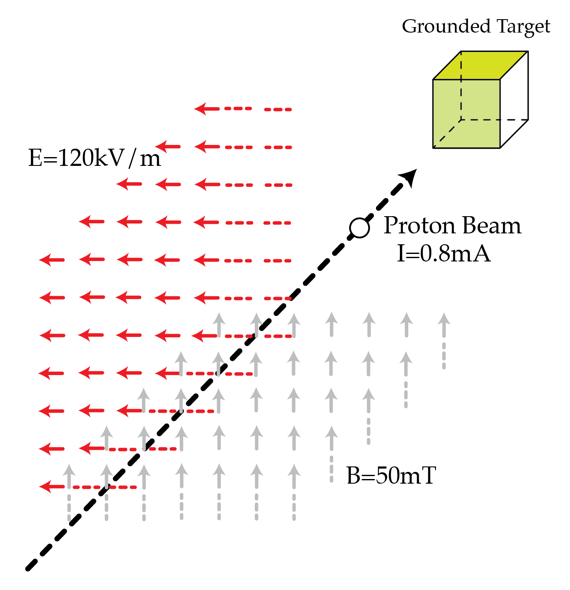Electricity and Magnetism

# Magnetic Fields: Level 2-4 ChallengesThe diagram above depicts two bar magnets suspended by string. If "N" labels one end of the magnet as magnetic north, then determine the magnetic pole labeled with "?".

An electric current of 1 Ampere is flowing along a horizontal wire. At $x=0$ the wire splits into a circle of radius 0.05 m and then comes back together at $x=0.1$ m. What is the magnitude in Tesla of the magnetic field in the middle of this loop of wire?Consider a standard cyclotron accelerator in which two semi-circular regions (dees) are connected to an AC voltage source which provides an electric field $E$ and a uniform vertical (out of the page) magnetic $B$ field inside cyclotron which is perpendicular to the electric field. The electric field switches such that is always points toward the dee the particle is not in.

A proton is released from rest such that it starts rotating in the cyclotron at radius $R$ and finally comes out from the slits of the cyclotron. The distance between the two semi-circular regions is $d$. Find the maximum number of turns proton take before coming out from slit's.

Details and Assumptions

${ { m }_{ p }=1.6\times { 10 }^{ -27 }\text{ kg}\\ { q }_{ p }=1.6\times { 10 }^{ -19 } C\\ B={ 10 }^{ -4 }\text{ T}\\ R=6\text{ m}\\ E=10\frac{\text{V}}{\text{m}}\\ d=10\text{ cm}\\ }$.

The charged particles from solar eruptions hit Earth mainly around the North and South poles (and cause auroras). This is because our Earth is similar to a big magnet. The Earth generates a magnetic field and this magnetic field funnels the charged particles towards the poles. In order to see an example of this funneling, we can think of the following problem:

Consider each magnetic pole separately (so there's only one pole in the problem). The magnetic field near a single pole is $\vec{B} = k\vec{r}/r^3=k\hat{r}/r^2$ where $\vec{r}$ is the radial vector point of the pole and the point of interest and $\hat{r}=\vec{r}/r$ is the radial unit vector. The sign of $k$ is opposite for the North and South poles. If there's an electric charge moving in that magnetic field, its trajectory is on a surface of a cone, i.e. a big funnel. Find the vertex angle (the angle between the axis and a line on the surface of the cone) in degrees with these given initial conditions: the distance between the charge and the pole is $r = 1~\mbox{m}$ and the velocity vector of the charge is $v = 2~\mbox{m/s}$ perpendicular to the line connecting the pole and the charge. We will consider a north pole and so let $k = 3~\mbox{T}\cdot\mbox{m}^2$. The charge of the particle is $q = 4~\mbox{C}$ and the mass is $m = 5~\mbox{kg}$.

Details and assumptions

• Hint: For anyone who has not taken electromagnetism yet, the force on the charge particle is given by the Lorentz force law: $\vec{F}=q\vec{v} \times \vec {B}$.A non-relativistic proton beam passes without deflection through a region where there are two transverse, mutually perpendicular, electric ($E=120 ~\textrm{kV/m}$) and magnetic ($B=50 ~\textrm{mT}$) fields. Then the beam strikes a grounded target. Find the force in Newtons with which the beam acts on the target if the beam current is $I=0.8 ~ \textrm{mA}$.

Assume that the collisions with the target are inelastic.
The proton's mass and charge are: $m_{p}=1.67 \times 10^{-27}\textrm{ kg}$ $e= 1.6 \times 10^{-19} C.$

×